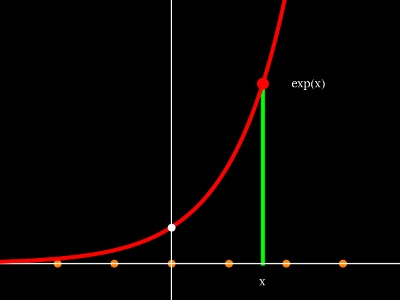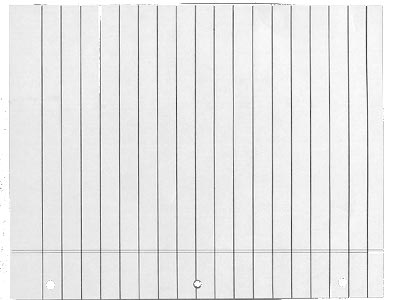# The exponentialThe function f(x) = 2x is an example of a exponential function. It grows very fast. We have for example f(0)=1, f(-2)=1/4, f(-10)=1/1024, f(10)=1024.If you fold a paper of thickness 0.1 mm once you get a stack 0.2mm. If you fold it again, you get a stack of thickness 0.4mm, After folding it 10 times you already have 10.2 cm.After folding 42 times, how thick is the stack? It is 242 * 0.1mm = 439804651 meters = 439805 km. Compare this with the average distance to the moon, which is 384403 km.After folding 100 times, how thick is the stack? It is 2100 * 0.1 mm = 1.27 * 1026 meters. We have seen distant clusters of galaxies 9.1 billion light years away. Today, Wednesday, one has just announced that galaxies 13.2 billion light years away have been seen. One light year is 24*365*3600*299792458 km = 9.1* 94607304725808000 m. The 100 times folded paper stack would no more fit into our observable universe.